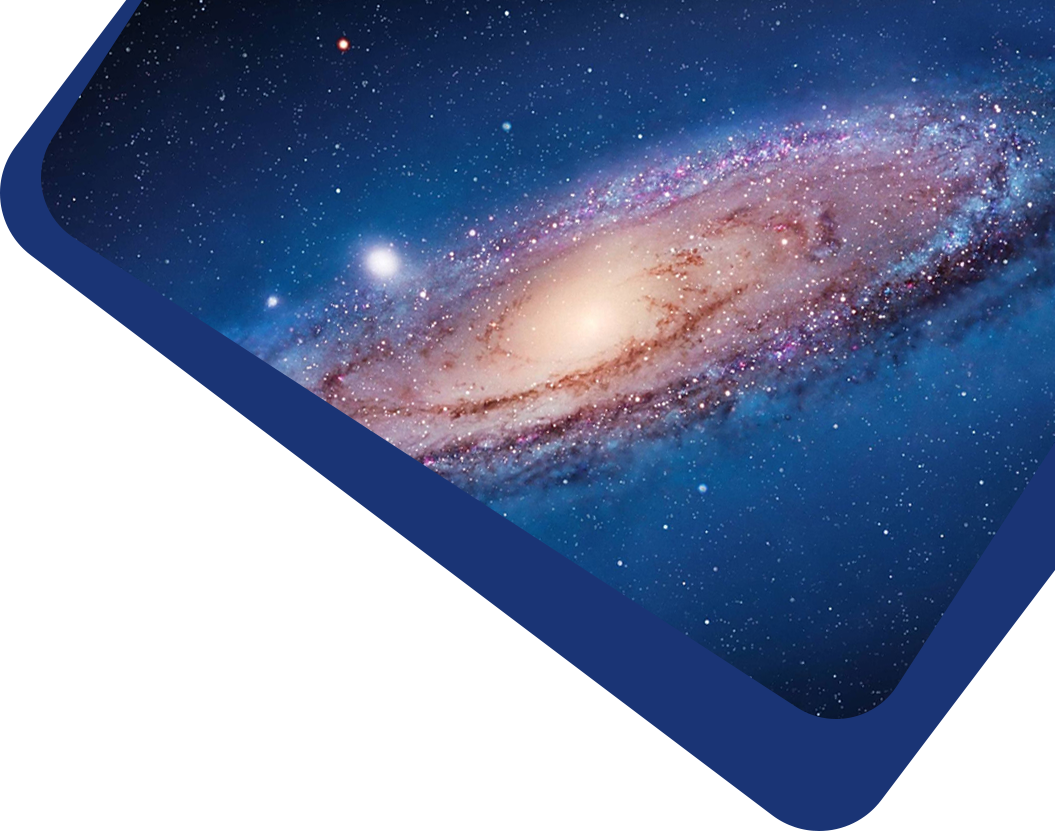Vol 22, No 11

## Revisiting the Evidence for an Intermediate-mass Black Hole in the Center of NGC 6624 with Simulations

#### Li-Chun Wang and Yi Xie

Abstract

The acceleration of LMXB 4U 1820-30 derived from its orbital-period derivative $$\dot{P}_{\rm b}$$ was supposed to be the evidence for an Intermediate-mass black hole (IMBH) in the Galactic globular cluster (GC) NGC 6624. However, we find that the anomalous $$\dot{P}_{\rm b}$$  is mainly due to the gravitational wave emission, rather than the acceleration in cluster potential. Using the standard structure models of GCs, we simulate acceleration distributions for pulsars in the central region of the cluster. By fitting the acceleration of J1823-3021A with the simulated distribution profiles (maximum values), it is suggested that an IMBH with mass M $$M\gtrsim 950_{-350}^{+550} M_{\odot}$$ may reside in the cluster center. We further show that the second period derivative $$\ddot{P}$$ of J1823-3021A is probably due to the gravitational perturbation of a nearby star.

Keywords

(stars:) pulsars: individual (J1823-3021A) – (Galaxy:) globular clusters: individual (NGC 6624) – stars: black holes

Full Text
Refbacks